• 文章目录一、固定分区分配1.1数据结构1.2 主函数 一、固定分区分配 1.1数据结构 class MemUsedTable{ // 内存 public: int ID; // 空闲分区的ID int size; // 空闲分区的大小 int address; // 空闲分区的开始...

要求

8 、固定式分区分配及可变式分区分配的存储管理方案设计与实现
【问题描述1】
设计一个固定式分区分配的存储管理方案，并模拟实现分区的分配和回收过程。
【设计要求1】
可以假定每个作业都是批处理作业，并且不允许动态申请内存。为实现分区的分配和回收，可以设定一个分区说明表，按照表中的有关信息进行分配，并根据分区的分配和回收情况修改该表。
【问题描述2】
设计一个可变式分区分配的存储管理方案，并模拟实现分区的分配和回收过程。
【设计要求2】
对分区的管理法至少是下面三种算法之一：
① 首次适应算法
② 循环首次适应算法
③ 最佳适应算法

• 首次使用算法，地址由高到低
• 最佳适应算法，内存空间由最小到大
• 最坏使用算法，内存空间由大到小

一、数据结构

• 建立内存分区链表
• 建立PCB进程需求链表

class MemUsedTable {   // 内存
public:
int ID;  // 空闲分区的ID
int size;  // 空闲分区的大小
int state;   // 0 表示未使用， 1表示使用
int workID;

MemUsedTable* next;  // 下一个的指针

MemUsedTable() {
this->ID = 0;
this->size = 0;
this->state = 0;
this->workID = 0;
this->next = NULL;
}

};

class PCB {
// 进程的数据结构
public:
int ID;  // PCB的ID
int size; // PCB需要的内存大小
int runState;   // 运行状态  0 表示未获得内存 1 表示获得内存
PCB* next;

PCB() {
this->ID = 0;
this->size = 0;
this->runState = 0;  //
this->next = NULL;   // 指向下一个
}

};

一、固定分区分配

一.1 最佳适应算法

#include<iostream>
#include<cstring>
#include<cstdlib>
#include<cmath>

using namespace std;

class MemUsedTable {   // 内存
public:
int ID;  // 空闲分区的ID
int size;  // 空闲分区的大小
int state;   // 0 表示未使用， 1表示使用
int workID;

MemUsedTable* next;  // 下一个的指针

MemUsedTable() {
this->ID = 0;
this->size = 0;
this->state = 0;
this->workID = 0;
this->next = NULL;
}

};

class PCB {
// 进程的数据结构
public:
int ID;  // PCB的ID
int size; // PCB需要的内存大小
int runState;   // 运行状态  0 表示未获得内存 1 表示获得内存
PCB* next;

PCB() {
this->ID = 0;
this->size = 0;
this->runState = 0;  //
this->next = NULL;   // 指向下一个
}

};

void dispPCBInfo(PCB*& pcbQ);         //  展示PCB链表的信息

MemUsedTable* sortMem(MemUsedTable* memReady, int flag);   // 对内存进行排序

PCB* inputPCB(PCB* pcbQ);      //  输入PCB块的信息
int label, int size);  // 单个内存的分配

MemUsedTable* allocateMems(MemUsedTable*& memReady, PCB*& pcbQ); // 对PCB进行分区

bool checkPCBEmpty(PCB* pcbQ);    // 检测PCB链表是否都在内存中执行

MemUsedTable* recoverMem(MemUsedTable* memReady, PCB* pcbQ);   // 回收内存
// ============================= //

cout << endl;

while (p != NULL) {
cout << "MEM->ID: " << p->ID << ", 大小: " << p->size << "B, 分配状态： " << p->state << ", 开始地址："
if (p->workID != 0) {
cout << ", 已分配的作业ID: " << p->workID << endl;
}
else {
cout << ", 未分配任何作业" << endl;
}
p = p->next;
}

cout << endl;

}

int cnt = 0;

MemUsedTable* p = memReady;  // NULL 指针

while (p != NULL) {
cnt++;
p = p->next;
}
return cnt;
}

// 输入内存大小的个数

// Notes：参数传入 指针时 不是全局的指针

/**
* 内存的大小以字节为单位
*
*/

int address = 0;   // 内存从0开始
int id = 0;    //  ID号从0开始
MemUsedTable* last = NULL;

// 输入内存
cout << "请输入现有内存的大小:(起始地址从0开始)" << endl;
int size;
cin >> size;

int MemSize = pow(2, size);

cout << "输入的内存的大小为: " << MemSize << "B" << endl;

// 进行分区，输入分区的块数，以及内存的大小， 2指数倍数

int num;
cout << "输入内存的块数: " << endl;
cin >> num;

for (int i = 0; i < num; ++i) {

cout << "第" << i << "号" << "内存, 输入内存的大小, 2的指数" << endl;
int n;  // 输入占用内存的大小， 2的指数
cin >> n;
// n 的判断 n 的范围在 0 - MemSize之间
int size = pow(2, n);

while (size >= MemSize) {
cout << "输入的n太大, 重新输入!" << endl;
cin >> n;
size = pow(2, n);
}

cout << "第" << i << "号,内存块的大小为: " << size << "B" << endl << endl;

MemUsedTable* mp = new MemUsedTable();
mp->ID = i;
mp->size = size;
mp->state = 0;  // 0表示未占用

//  调整全局的信息
MemSize -= size;

// 链接到链表后
// 没有表格的时候
}
else {
// 加入链表中
last->next = mp;
last = mp;
}

}
}

MemUsedTable* sortMem(MemUsedTable* memReady, int flag) {

if (p1 == NULL) {
cout << "链表为空" << endl;
return NULL;
}

if (p1->next == NULL) {
cout << "只有一个节点不用排序" << endl;
return NULL;
}

if (flag == 1) {
// 最佳适应算法
while (p1->next != NULL) {
// 指向下一个
MemUsedTable* p2 = p1->next;

while (p2 != NULL) {
if (p1->size > p2->size) {
// 交换指针
//				MemUsedTable tmp = *p1;
//				*p1 = *p2;
//				*p2 = temp;
//
//				tmp.next = p1;
//				p1->next = p2->next;
//				p2->next = tmp.next;

int ID = p1->ID;
int size = p1->size;
int workID = p1->workID;
int state = p1->state;

p1->ID = p2->ID;
p1->size = p2->size;
p1->workID = p2->workID;
p1->state = p2->state;

p2->ID = ID;
p2->size = size;
p2->workID = workID;
p2->state = state;

}

p2 = p2->next;
}
p1 = p1->next;
}
}

else if (flag == 2) {
// 最坏适应算法
while (p1->next != NULL) {
// 指向下一个
MemUsedTable* p2 = p1->next;

while (p2 != NULL) {
if (p1->size < p2->size) {
// 交换指针
//				MemUsedTable tmp = *p1;
//				*p1 = *p2;
//				*p2 = temp;
//
//				tmp.next = p1;
//				p1->next = p2->next;
//				p2->next = tmp.next;

int ID = p1->ID;
int size = p1->size;
int workID = p1->workID;
int state = p1->state;

p1->ID = p2->ID;
p1->size = p2->size;
p1->workID = p2->workID;
p1->state = p2->state;

p2->ID = ID;
p2->size = size;
p2->workID = workID;
p2->state = state;

}

p2 = p2->next;
}
p1 = p1->next;
}

}

else if (flag == 3) {
// 首次使用算法， 不排序
}
}

void dispPCBInfo(PCB*& pcbQ) {
PCB* p = pcbQ;

cout << endl;

while (p != NULL) {
cout << "PCB->ID: " << p->ID << ", PCB运行状态: " << p->runState << ", PCB大小： " <<
p->size << "B, " << ", PCB运行地址: " << p->address << endl;
p = p->next;
}

cout << endl;

}

PCB* inputPCB(PCB* pcbQ) {
/**
* 作业不排序
*/
cout << "输入作业的个数" << endl;

int num;
PCB* last = NULL;
cin >> num;

for (int i = 1; i <= num; ++i) {
PCB* np = new PCB();
np->ID = i;

cout << "输入PCB的大小" << endl;

int pcbSize;
cin >> pcbSize;

np->size = pcbSize;

if (pcbQ == NULL) {
pcbQ = np;
last = pcbQ;
}
else {
last->next = np;
last = np;
}
}

return pcbQ;

}

MemUsedTable* allocateMem(MemUsedTable*& memReady, int label, int size) {
/**
* 分配内存给作业 label 为作业号， size为分配内存的大小
*/

while (p != NULL) {
if (p->size >= size) {

if (p->state != 1) {
p->state = 1;
p->workID = label;
return p;
}
}

p = p->next;
}
return NULL;

}

MemUsedTable* allocateMems(MemUsedTable*& memReady, PCB*& pcbQ) {
/**
* 1. 循环作业进行分配
* 2. 修改信息
*/
PCB* p = pcbQ;

while (p != NULL) {

if (p->runState != 1) {
MemUsedTable* allocated_mem = allocateMem(memReady, p->ID, p->size);

if (allocated_mem != NULL) {
cout << "分配成功: PCB_ID: " << p->ID << " 分配的内存块ID: " << allocated_mem->ID << endl;
p->runState = 1;

}
}
p = p->next;

}

}

bool flag = true;    // 假设内存已经全部释放

while (p != NULL) {
if (p->state == 1) {
return false;
}

p = p->next;

}
return flag;

}

bool checkPCBEmpty(PCB* pcbQ) {
PCB* p = pcbQ;

while (p != NULL) {
if (p->runState == 0) {
return false;
}
p = p->next;
}

return true;
}

MemUsedTable* recoverMem(MemUsedTable* memReady, PCB* pcbQ) {
/**
* 收回固定分配的内存
* 输入要收回分区的个数，输入要收回分区的ID号，
* 根据ID号释放内存， 更改内存块的状态
*
*/
int num;

while (1) {

cout << endl << endl;

cout << "输入要释放的内存块号" << endl;
int memBlock;
cin >> memBlock;

cout << "作业已经调度完, 并且内存以释放完" << endl;
dispPCBInfo(pcbQ);
break;
}

while (p != NULL) {
if (p->ID == memBlock) {
if (p->state == 1) {
p->state = 0;   // 释放内存
p->workID = 0;   // 修改被占用的工作块
cout << "内存释放成功" << endl;
dispPCBInfo(pcbQ);
}
else if (p->state == 0) {
cout << "此内存块为空闲块" << endl;
}
}
p = p->next;
}

cout << "作业已经调度完, 并且内存以释放完" << endl;
dispPCBInfo(pcbQ);
break;
}

char flag;
cout << "是否继续释放内存 y/Y ->Yes, n/N ->No" << endl;
cin >> flag;

while (!(flag == 'Y' || flag == 'y' || flag == 'N' || flag == 'n')) {
cout << "输入 y/Y ->Yes, n/N ->No" << endl;
cin >> flag;
}

if (flag == 'y' || flag == 'Y') {
continue;
}
else if (flag == 'N' || flag == 'n') {
break;
}
}

cout << endl << "内存状态： " << endl;

}

int main() {
cout << "<<<<<<<<<<<<<<<<<<<<<< 固定分区， 最佳适应算法算法 >>>>>>>>>>>>>>>>>>>>>>>>>>>>" << endl;

MemUsedTable* memReady = NULL;  // 内存链表

MemUsedTable* sortmem = sortMem(mem, 1);

PCB* pcbQ = NULL;

PCB* input_pcb = inputPCB(pcbQ);

dispPCBInfo(input_pcb);

allocateMems(sortmem, input_pcb);

dispPCBInfo(input_pcb);

recoverMem(sortmem, input_pcb);

system("pause");

return 0;
}

/**
测试  Case : 1
10
4
5
6
5
4
6
20
10
20
20
30
20

*/

二、可变分区分配方式

二、1 可变分区-最佳适应算法

#include<iostream>
#include<cstring>
#include<cstdlib>
#include<cmath>

using namespace std;

class MemUsedTable {   // 内存
public:
int ID;  // 空闲分区的ID
int size;  // 空闲分区的大小
int state;   // 分区的状态 0 未分配 1 分配
int workID;
MemUsedTable* pre;   // 前一个指针
MemUsedTable* next;  // 下一个指针

MemUsedTable() {
this->ID = 0;
this->size = 0;
this->state = 0;  // 未使用
this->workID = 0;
this->next = NULL;
this->pre = NULL;
}

};

class PCB {
// 进程的数据结构
public:
int ID;  // PCB的ID
int size; // PCB需要的内存大小
int runState;   // 运行状态
PCB* next;

PCB() {
this->ID = 0;
this->size = 0;
this->runState = 0;  // 0 未运行 1 运行
this->next = NULL;   // 指向下一个
}

};

void dispPCBInfo(PCB*& pcbQ);    // 展示PCB进程的信息

PCB* inputPCB(PCB* pcbQ);         // 输入进程PCB的信息，PCB的大小
MemUsedTable* sortMem(MemUsedTable* memReady, int flag);  // 对内存按照要求排序
// 1 最佳适应 2 最坏适应， 3 首次适应
bool checkPCBEmpty(PCB* pcbQ);          // 检测内存都已经得到分配，并且运行
MemUsedTable* allocateMem(MemUsedTable*& memReady, int label, int size);
// 分配单个进程PCB

MemUsedTable* allocateMems(MemUsedTable*& memReady, PCB*& pcbQ); // 对PCB链表进行内存分配

MemUsedTable* recoverMem(MemUsedTable* memReady, PCB* pcbQ);   // 回收内存

///====================================

cout << endl;

while (p != NULL) {
cout << "Mem->ID: " << p->ID << ", 大小: " << p->size << "B, 分配状态： " << p->state << ", 开始地址："
if (p->workID != 0) {
cout << ", 已分配的作业ID: " << p->workID << endl;
}
else {
cout << ", 未分配任何作业" << endl;
}
p = p->next;
}

cout << endl;

}

int cnt = 0;

MemUsedTable* p1 = memReady;  // NULL 指针

// 链表为空的时候
if (p1 == NULL) {
cout << "链表为空" << endl;
return 0;
}

while (p1 != NULL) {
cnt++;
p1 = p1->next;
}

return cnt;
}

// 输入内存大小的个数

// 输入内存
cout << "请输入现有内存的大小:(起始地址从0开始)" << endl;
int size;
cin >> size;

int MemSize = pow(2, size);

// 建立主内存块
MemUsedTable* mp = new MemUsedTable();
mp->ID = 0;
mp->size = MemSize;
mp->state = 0;  // 0表示未占用

cout << "输入的内存的大小为: " << MemSize << "B" << endl;

}

void dispPCBInfo(PCB*& pcbQ) {
PCB* p = pcbQ;

cout << endl;

while (p != NULL) {
cout << "PCB->ID: " << p->ID << ", PCB运行状态: " << p->runState << ", PCB大小： " <<
p->size << "B, " << ", PCB运行地址: " << p->address << endl;
p = p->next;
}

cout << endl;

}

PCB* inputPCB(PCB* pcbQ) {
/**
* 作业不排序
*/
cout << "输入作业的个数" << endl;

int num;
PCB* last = NULL;
cin >> num;

for (int i = 1; i <= num; ++i) {
PCB* np = new PCB();
np->ID = i;

cout << "输入PCB的大小" << endl;

int pcbSize;
cin >> pcbSize;

np->size = pcbSize;

if (pcbQ == NULL) {
pcbQ = np;
last = pcbQ;
}
else {
last->next = np;
last = np;
}
}

return pcbQ;

}

MemUsedTable* sortMem(MemUsedTable* memReady, int flag) {

if (p1 == NULL) {
cout << "链表为空" << endl;
return NULL;
}

if (p1->next == NULL) {
cout << "只有一个节点不用排序" << endl;
}

if (flag == 1) {
// 最佳适应算法
while (p1->next != NULL) {
// 指向下一个
MemUsedTable* p2 = p1->next;

while (p2 != NULL) {
if (p1->size > p2->size) {
// 交换指针
//				MemUsedTable tmp = *p1;
//				*p1 = *p2;
//				*p2 = temp;
//
//				tmp.next = p1;
//				p1->next = p2->next;
//				p2->next = tmp.next;

int ID = p1->ID;
int size = p1->size;
int workID = p1->workID;
int state = p1->state;

p1->ID = p2->ID;
p1->size = p2->size;
p1->workID = p2->workID;
p1->state = p2->state;

p2->ID = ID;
p2->size = size;
p2->workID = workID;
p2->state = state;

}

p2 = p2->next;
}
p1 = p1->next;
}
}

else if (flag == 2) {
// 最坏适应算法
while (p1->next != NULL) {
// 指向下一个
MemUsedTable* p2 = p1->next;

while (p2 != NULL) {
if (p1->size < p2->size) {
// 交换指针
//				MemUsedTable tmp = *p1;
//				*p1 = *p2;
//				*p2 = temp;
//
//				tmp.next = p1;
//				p1->next = p2->next;
//				p2->next = tmp.next;

int ID = p1->ID;
int size = p1->size;
int workID = p1->workID;
int state = p1->state;

p1->ID = p2->ID;
p1->size = p2->size;
p1->workID = p2->workID;
p1->state = p2->state;

p2->ID = ID;
p2->size = size;
p2->workID = workID;
p2->state = state;

}

p2 = p2->next;
}
p1 = p1->next;
}

}

else if (flag == 3) {
// 首次使用算法， 不排序
}
}

bool flag = true;    // 假设内存已经全部释放

while (p != NULL) {
if (p->state == 1) {
return false;
}

p = p->next;

}
return flag;

}

bool checkPCBEmpty(PCB* pcbQ) {
PCB* p = pcbQ;

while (p != NULL) {
if (p->runState == 0) {
return false;
}
p = p->next;
}

return true;
}

MemUsedTable* allocateMem(MemUsedTable*& memReady, int label, int size) {
/**
* 分配内存给作业 label 为作业号， size为分配内存的大小
*/

while (p != NULL) {

if (p->size >= size) {
// 未被占用
if (p->state != 1) {
p->state = 1;
p->workID = label;

// 割掉一个分区
if (p->size > size) {
MemUsedTable* mp = new MemUsedTable();

int splitSize = p->size - size;

// 修改分区信息
mp->ID = ID;
mp->size = splitSize;
mp->state = 0;
mp->workID = 0;

// 添加到链表中
if (p->next != NULL) {
MemUsedTable* nextP = p->next;
mp->pre = p;
p->next = mp;

nextP->pre = mp;
mp->next = nextP;
}
else {
// 只有一个分区的时候与
p->next = mp;
mp->pre = p;
}

}

// 修改p的状态
p->size = size;

return p;
}

}

p = p->next;
}
return NULL;

}

MemUsedTable* allocateMems(MemUsedTable*& memReady, PCB*& pcbQ) {

PCB* p = pcbQ;

while (p != NULL) {

if (p->runState != 1) {
MemUsedTable* allocated_mem = allocateMem(memReady, p->ID, p->size);

if (allocated_mem != NULL) {
cout << "分配成功: PCB_ID: " << p->ID << " 分配的内存块ID: " << allocated_mem->ID << endl;
p->runState = 1;

}
}
p = p->next;

}
}

MemUsedTable* recoverMem(MemUsedTable* memReady, PCB* pcbQ) {
/**
* 动态回收内存
* 输入要释放内存的个数
* 动态回收的情形
*   1. 上一个是空闲分区， 与上一个合并，开始的地址为上一个空闲分区
*   2. 下一个是空闲分区， 与下一个合并，开始的地址为这个内存区的地址
*   3. 上一个与下一个都是空闲分区， 与上下合并，内存分区减少一个， 开始第一位号小的那个
*   4. 没有相邻的时候， 放到队列中
*/

while (1) {

cout << endl << endl;

// 检查内存为空
cout << "作业已经调度完, 并且内存以释放完" << endl;
dispPCBInfo(pcbQ);
break;
}

cout << "输入要释放的内存块号" << endl;
int memBlock;
cin >> memBlock;

while (memBlock >= memLength) {
cout << "内存块号越界!, 请重新输入" << endl;
cin >> memBlock;
}

while (p != NULL) {
if (p->ID == memBlock) {
if (p->state == 1) {

// 判断前后分区
if (p->pre != NULL && p->next != NULL) {
if (p->pre->state == 0 && p->next->state == 0) {
// 前后分区空闲

cout << endl << "前为空，后为空" << endl << endl;
// 修改信息
p->pre->size += p->size + p->next->size;

// 确定地址

// 指针移动 三个分区合并
p->pre->next = p->next->next;

if (p->next->next != NULL) {
p->next->next->pre = p->pre;
}

// delete p->next;
// delete p;

}
else if (p->pre->state == 0 && p->next->state == 1) {
// 前一个空闲
cout << endl << "前为空，后不为空" << endl << endl;

p->pre->size += p->size;

// 修改指针
p->pre->next = p->next;
p->next->pre = p->pre;
// delete p;

}
else if (p->pre->state == 1 && p->next->state == 0) {

cout << endl << "前不为空，后为空" << endl << endl;

// 修改状态
p->size += p->next->size;

p->state = 0;
p->workID = 0;

// 从后指向前， 在从前指向后

// 修改指针
if (p->next->next != NULL) {

p->next->next->pre = p;
// p->next->pre = NULL;

p->next = p->next->next;
// p->next->next  = NULL;

}

// 后面的内存只有一个的时候
if (p->next->next == NULL) {
p->next = NULL;
}

// delete p->next;

}
else if (p->pre->state == 1 && p->next->state == 1) {

cout << endl << "前不为空，后不为空" << endl << endl;

p->state = 0;
p->workID = 0;
}
}

else if (p->pre == NULL && p->next != NULL) {
if (p->next->state == 0) {

cout << endl << "前为NULL, 后为空" << endl << endl;

// 修改状态
p->size += p->next->size;

p->state = 0;
p->workID = 0;

// 修改指针
if (p->next->next != NULL) {

p->next->next->pre = p;
// p->next->pre = NULL;

p->next = p->next->next;
// p->next->next  = NULL;

}

// MemUsedTable* tmp = p->next;

// 后面的内存只有一个的时候
if (p->next->next == NULL) {
p->next = NULL;
}

// delete tmp;

}
else {
cout << endl << "前为NULL, 后不为空" << endl << endl;
p->state = 0;
p->workID = 0;

}
}

else if (p->next == NULL && p->pre != NULL) {
if (p->pre->state == 0) {

cout << endl << "前为空，后为NULL" << endl << endl;

p->pre->size += p->size;

// 移动指针
p->pre->next = p->next;  // 为NULL

// delete p;
}
else {
cout << endl << "前不为空，后为NULL" << endl << endl;

p->state = 0;
p->workID = 0;

}
}

cout << "内存释放成功" << endl;
// 释放完之后 再次分配

dispMemInfo(sortedMem);
dispPCBInfo(pcbQ);
allocateMems(sortedMem, pcbQ);
dispMemInfo(sortedMem);
dispPCBInfo(pcbQ);
}
else if (p->state == 0) {
cout << "此内存块为空闲块" << endl;
}

break;
}

p = p->next;
}

// 检查内存为空

cout << "作业已经调度完, 并且内存以释放完" << endl;
dispPCBInfo(pcbQ);
break;
}

// 合法的输入
char flag;
cout << "是否继续释放内存 y/Y ->Yes, n/N ->No" << endl;
cin >> flag;

while (!(flag == 'Y' || flag == 'y' || flag == 'N' || flag == 'n')) {
cout << "输入 y/Y ->Yes, n/N ->No" << endl;
cin >> flag;
}

if (flag == 'y' || flag == 'Y') {
continue;
}
else if (flag == 'N' || flag == 'n') {
break;
}
}

// 最后展示内存的状态
cout << endl << "内存状态： " << endl;

}

int main() {

dispMemInfo(mem);

PCB* pcbQ = NULL;

pcbQ = inputPCB(pcbQ);

dispPCBInfo(pcbQ);

MemUsedTable* allocate_mem = allocateMems(mem, pcbQ);

dispMemInfo(allocate_mem);
dispPCBInfo(pcbQ);

recoverMem(allocate_mem, pcbQ);

return 0;
}

/**

测试 Case1 :
7
3
50
60
30

*/

展开全文数据结构
• 文章目录前言知识总览单一连续分配固定分区分配动态分区分配1. 系统要用什么样的数据结构记录内存的使用情况?2. 当很多个空闲分区都能满足需求时，应该选择哪个分区进行分配?3. 如何进行分区的分配与回收操作?分配...

前言

此篇文章是我在B站学习时所做的笔记，大部分图片都是课件老师的PPT，方便复习用。此篇文章仅供学习参考。

提示：以下是本篇文章正文内容

知识总览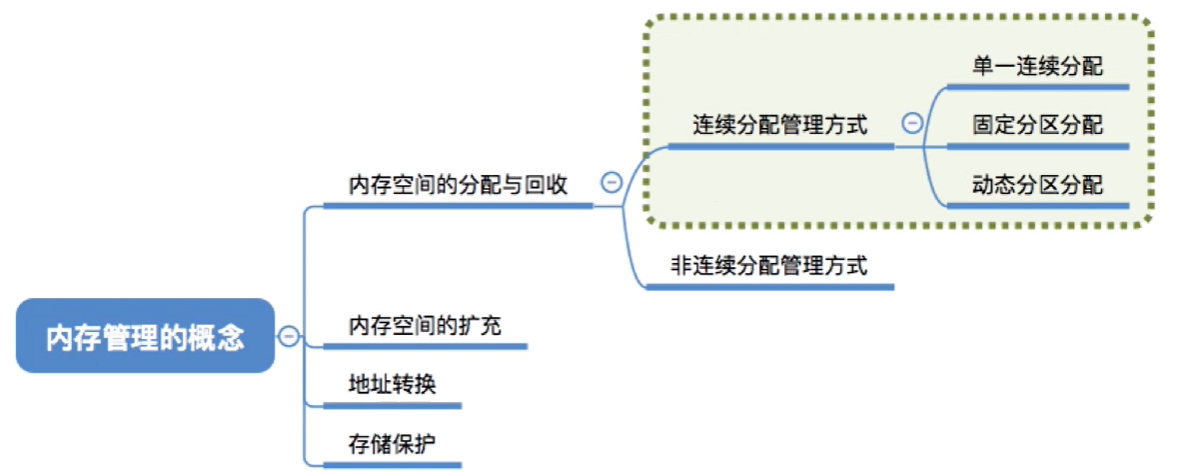连续分配：指为用户进程分配的必须是一个连续的内存空间

单一连续分配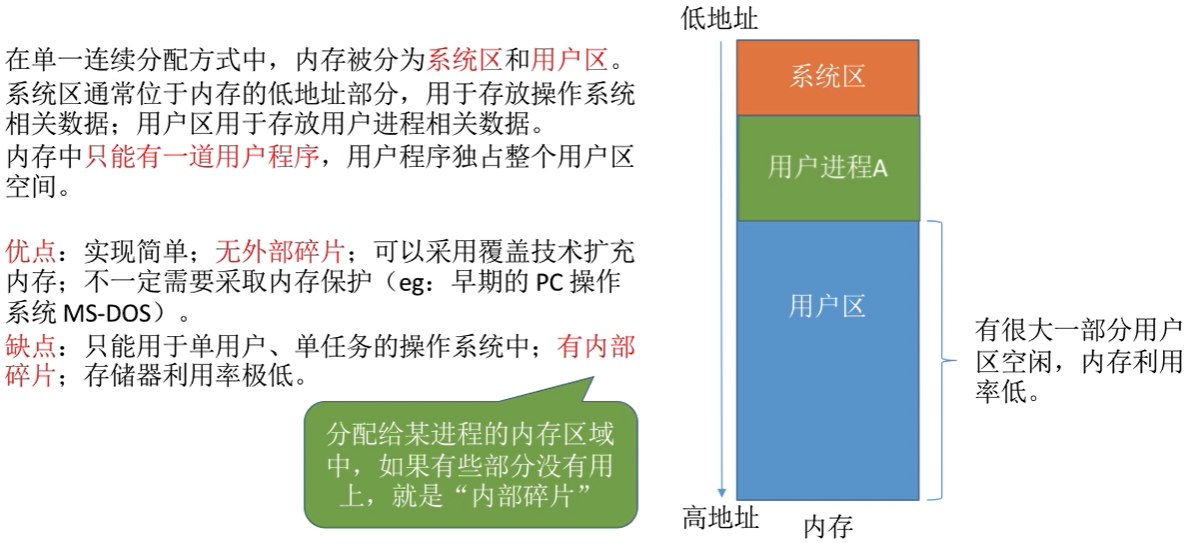重点！！！
MS—DOS 的存储管理采用了单一连续分配方式
只能用于单用户、单任务的操作系统！！！ 内存中只能有一道用户程序，用户程序独占整个用户区空间。
缺点：会产生内部碎片

解说
由于整个系统当中同一时刻只会有一个用户程序的运行，所以采用这种分配方式的系统当中，不一定需要采用内存保护，有的系统当中，它也会设置那种越界检查了一些机制，但是像早期的个人操作系统，微软的MS-DOS系统就没有采用这种内存保护的机制，因为系统中只会运行一个用户程序，那么即使这个用户程序出问题了，那也只会影响用户进程本身，或者说即使这个用户程序越界，把操作系统的数据损坏了，那这个数据一般来说也可以通过重启计算机就可以很方便地这进行修复，所以说采用单一连续分配的系统当中，不一定采取内存保护，那这也是它的优点呐，另一方面这个方式的缺点也很明显，就是只适用于单用户，单任务的操作系统，它并不支持多道程序并发运行，并且这种方式会产生内部碎片，那所谓的内部碎片就是指我们分配给某一个进程，或者说程序的内存区间当中，如果有重要部分没有被用上，那这就是所谓的内部碎片，像这个例子当中，本来整个用户区都是分配给这个用户进来的，但是有这么大一块，它是空闲的，暂时没有用起来，那本来给这个进程分配了，但是这个进程没有用上了这一部分内存区就是所谓的内部碎片，所以这个方式也会导致存储器的利用率很低

固定分区分配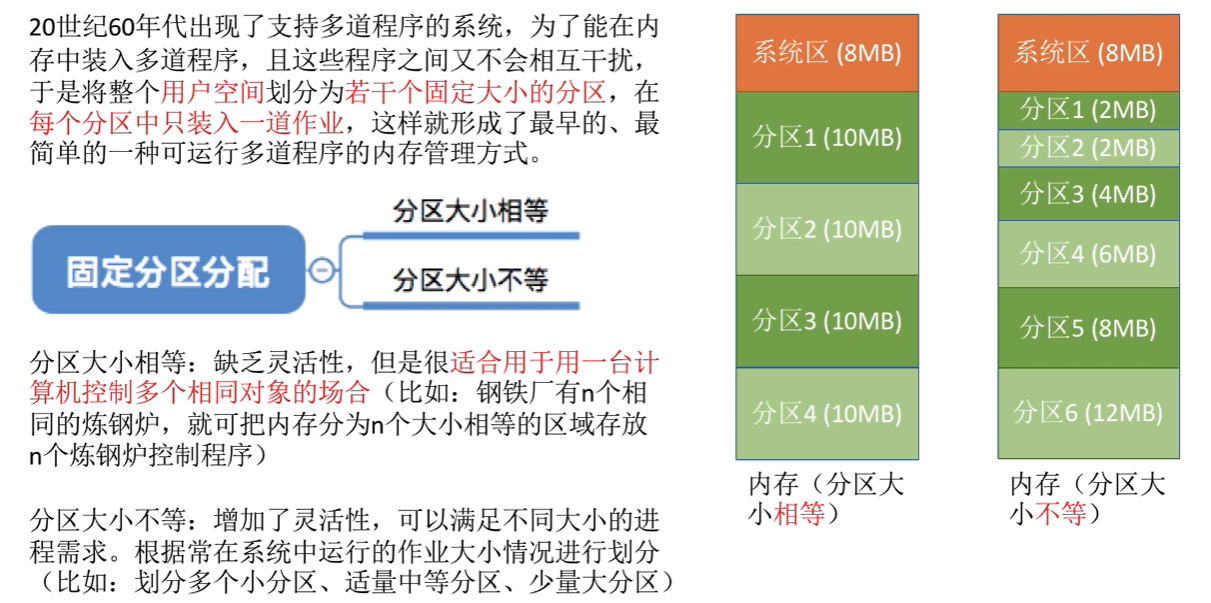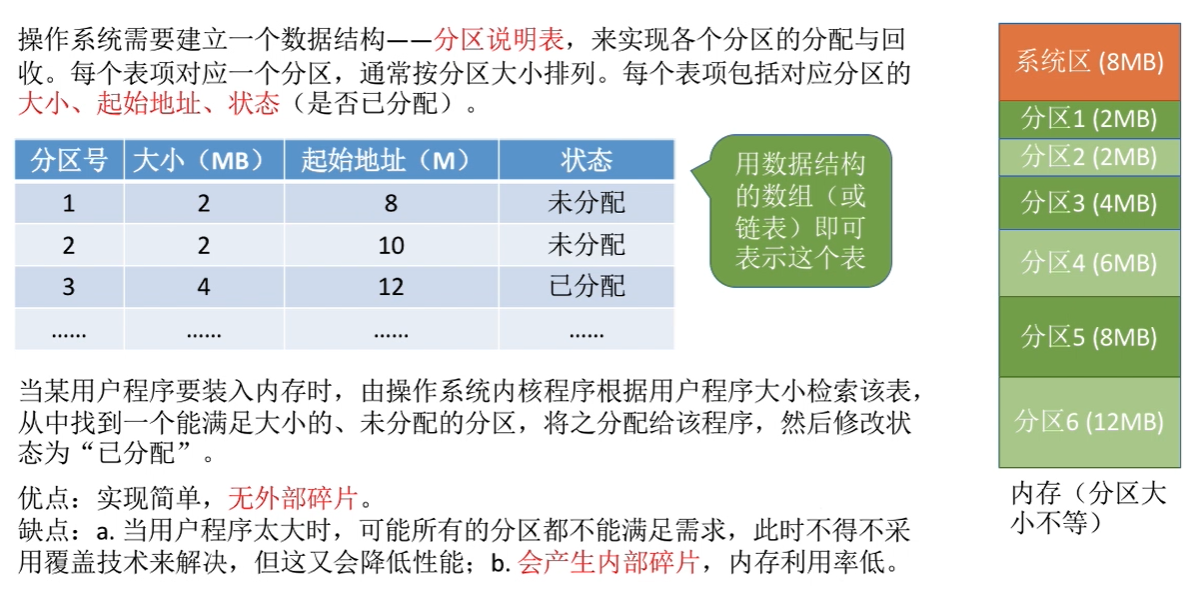无外部碎片，会产生内部碎片

动态分区分配

动态分区分配又称为可变分区分配。这种分配方式不会预先划分内存分区，而是在进程装入内存时，根据进程的大小动态地建立分区，并使分区的大小正好适合进程的需要。因此系统分区的大小和数目是可变的。(eg：假设某计算机内存大小为64MB，系统区8MB，用户区共56 MB.….)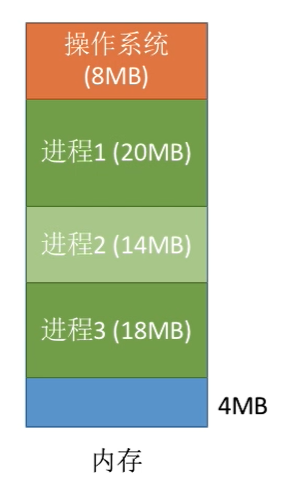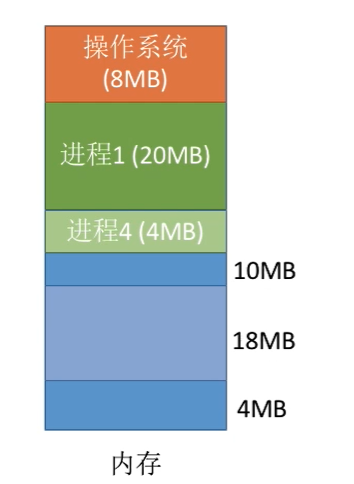1. 系统要用什么样的数据结构记录内存的使用情况?

两种数据结构：空闲分区表空闲分区链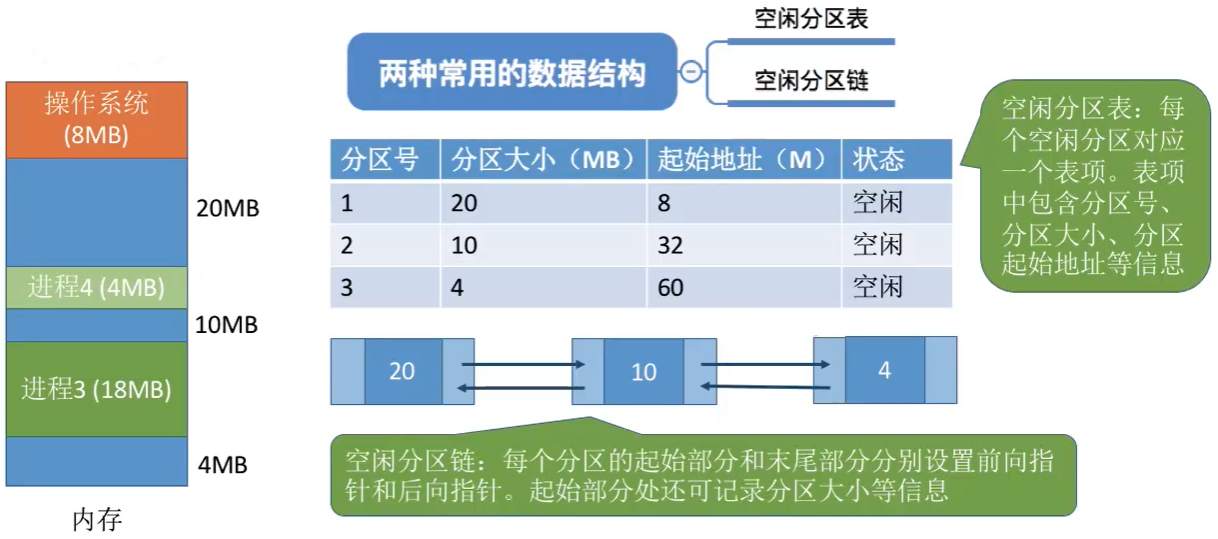2. 当很多个空闲分区都能满足需求时，应该选择哪个分区进行分配?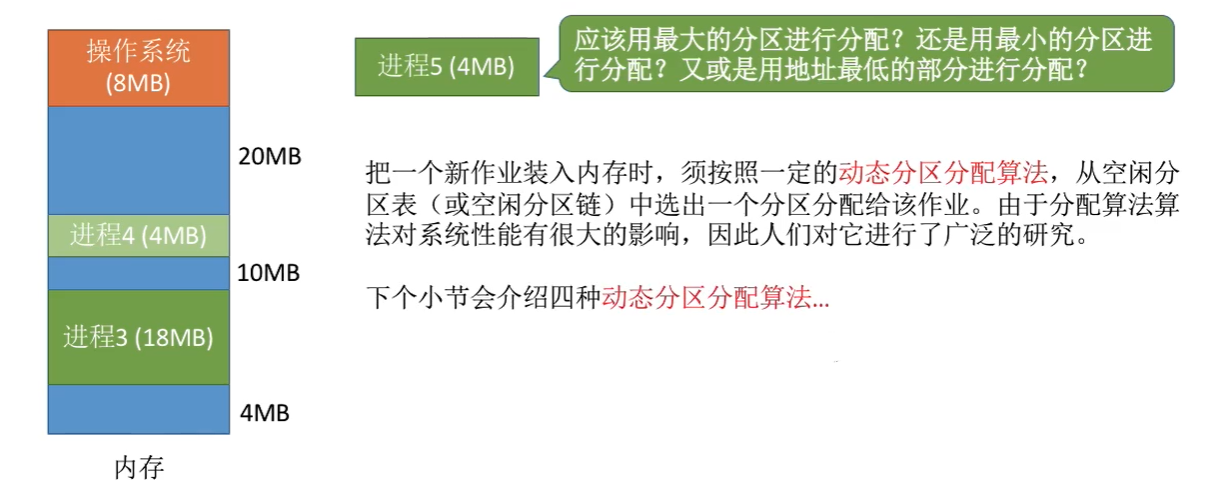3. 如何进行分区的分配与回收操作?

假设系统采用的数据结构是“空闲分区表”…
如何回收?
空闲分区表为例

分配方式一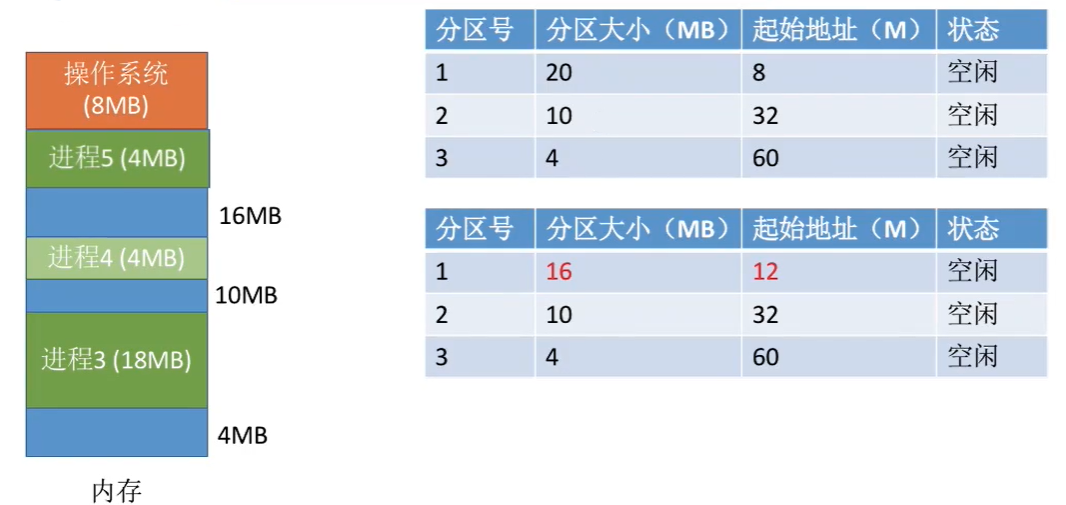分配方式二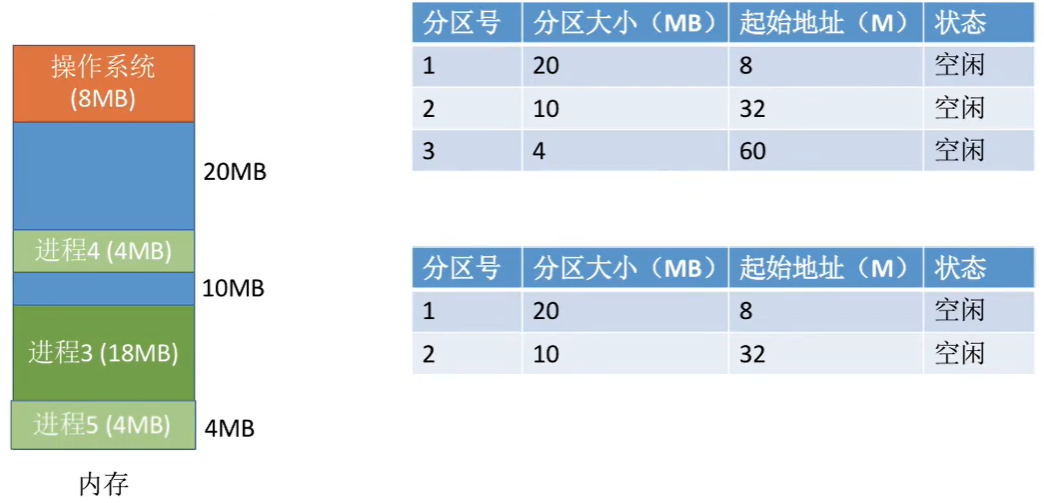解说
还是相同的例子，有一个进程五需要4MB（四兆字节），那如果说我们采用了某种分配算法，最后决定把这4M字节的空闲分区分配给进程5，那么本来这个空闲分区（分区号3，分区大小4）的大小，就和此次申请的这个内存空间大小是相同的，所以如果把这个分区空闲分区全部分配给这个进程的话，那么显然空闲分区的数量会-1，所以我们需要把这个分区对应的这个表项给删除，那如果说我们采用的是空闲分区链的话，那我们就是需要把其中的某一个而空闲分区链的节点给删掉。那这是分配的时候可能会遇到的两种情况。

回收情况一：回收区的后面有一个相邻的空闲分区

合并前的分区：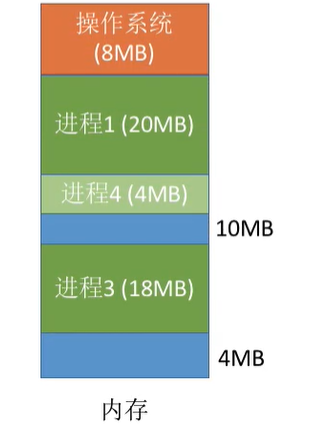合并后：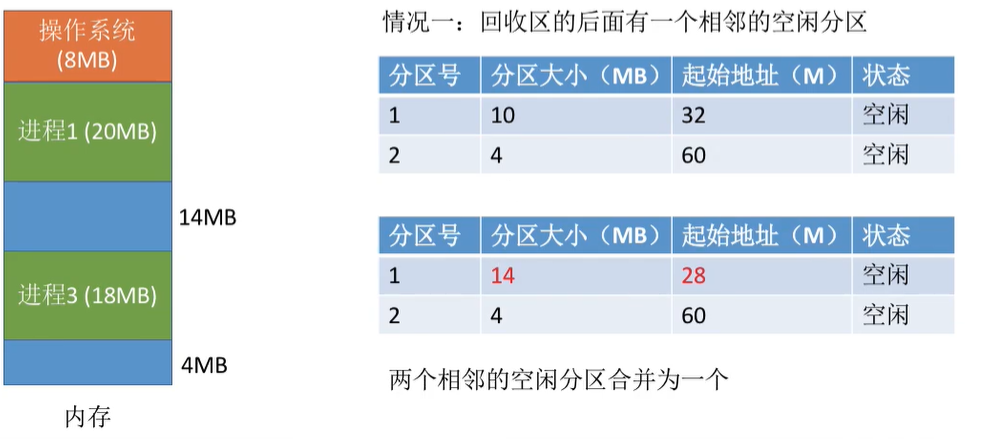回收情况二：回收区的前面有一个相邻的空闲分区

合并前：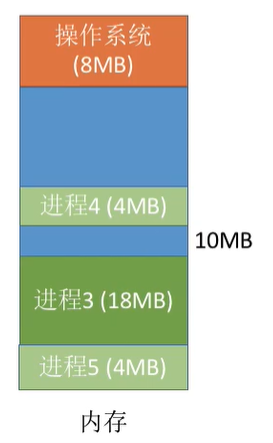合并后：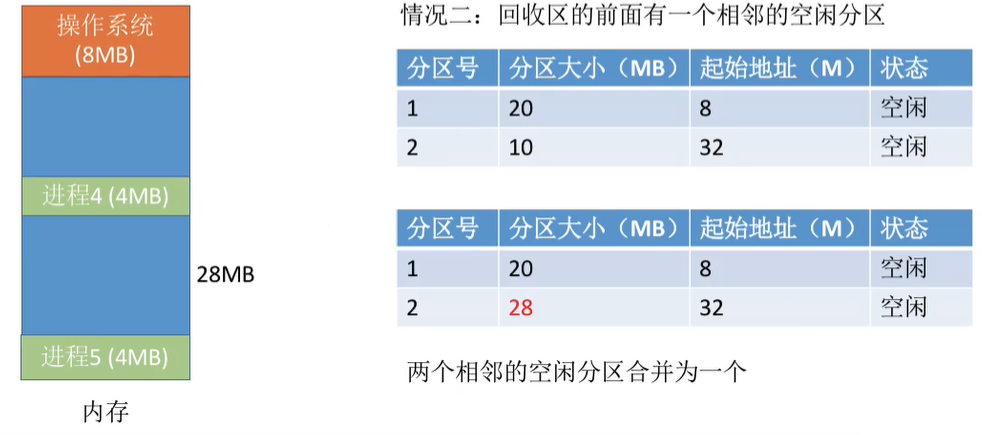回收情况三：回收区的前、后各有一个相邻的空闲分区

合并前：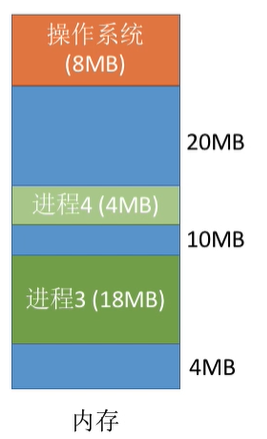合并后：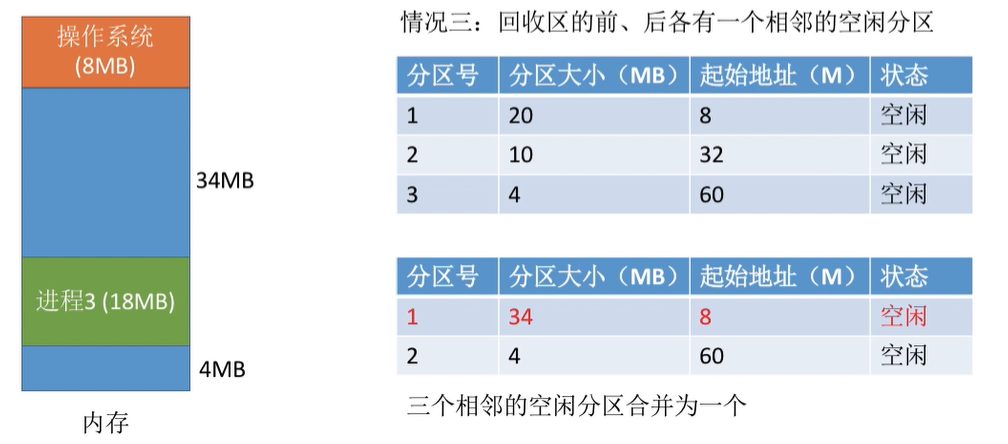回收情况四：回收区的前、后都没有相邻的空闲分区

合并前：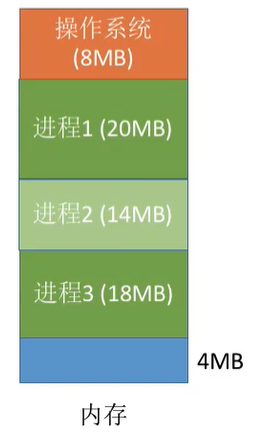合并后：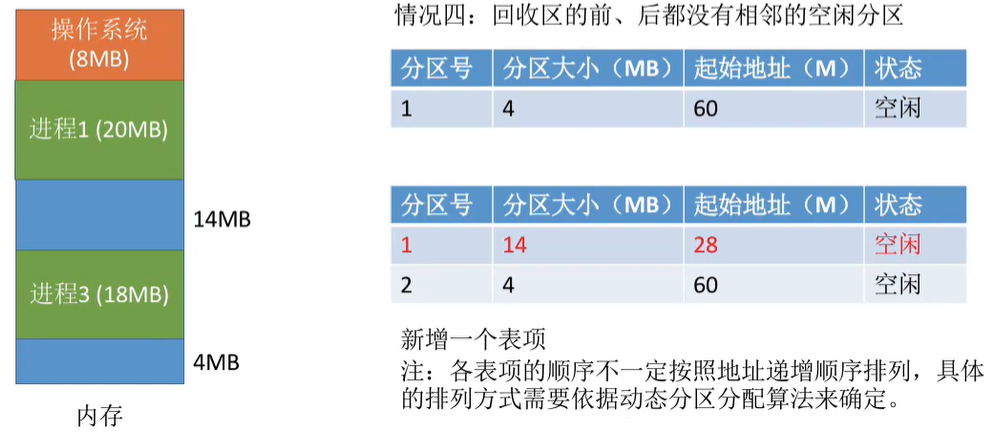碎片问题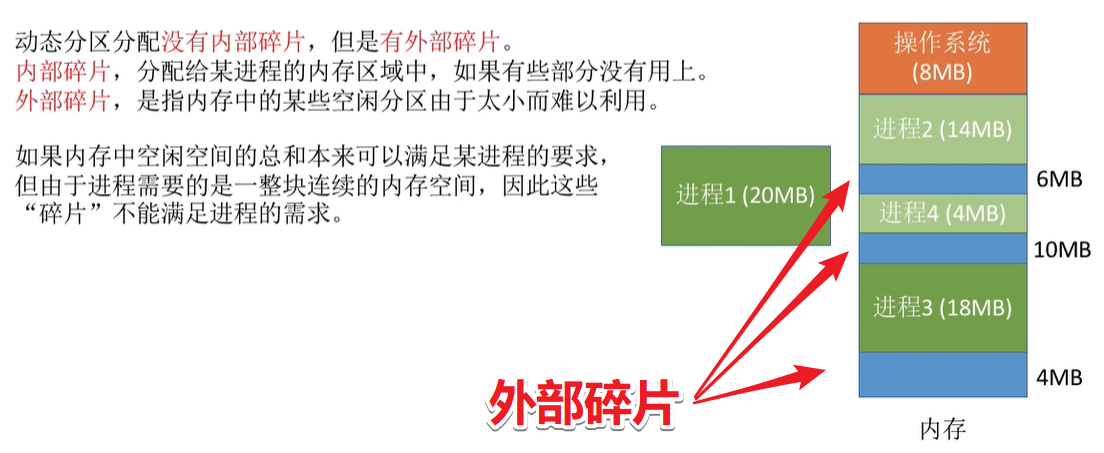重点！！！
动态分区分配没有内部碎片，但是有外部碎片。

可以通过紧凑（拼凑，Compaction）技术来解决外部碎片。即将各个进程挪位，挪出一个连续的空闲区域出来👇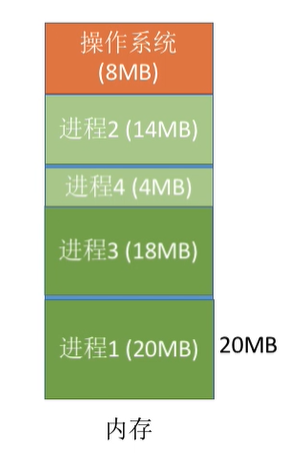4. 思考动态分区分配应使用哪种装入方式？“紧凑”之后需要做什么处理?

：①显然之前介绍的三种装入方式当中，动态重定位的方式，其实是最方便实现进程在内存当中移动位置这件事，所以我们应该采用的是 动态重定位 的方式。
②紧凑之后，需要各个进程的起始地址给修改掉，进程的起始地址信息一般存放在进程对应的PCB当中，当进程要上CPU运行之前，会把进程的起始地址那个信息放到重定位寄存器里或者叫基址寄存器里。

知识回顾与重要考点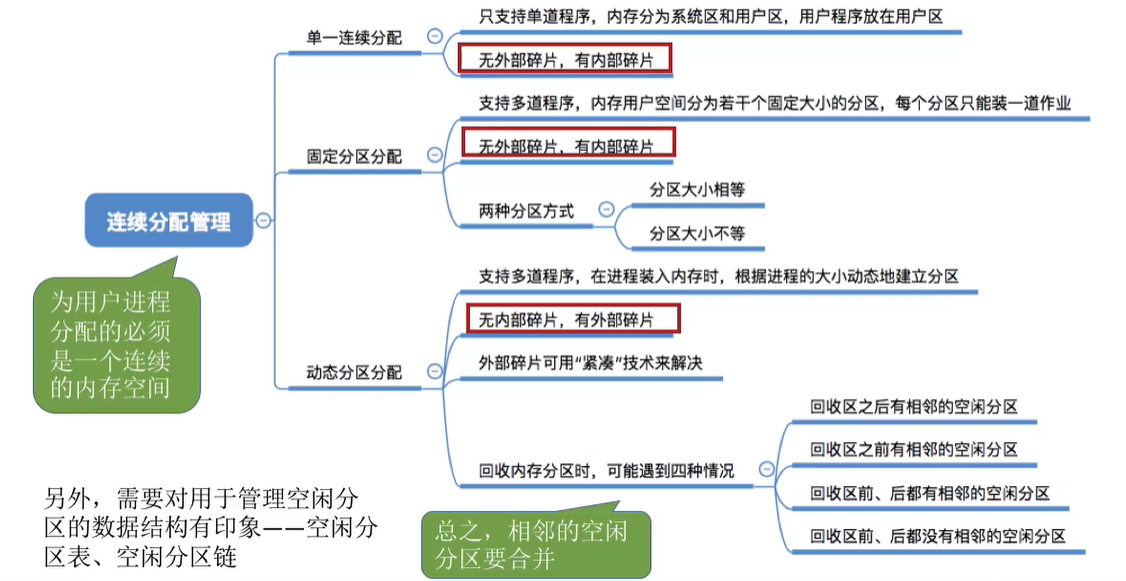展开全文操作系统 连续分配管理方式
• 计算机操作系统-固定分区分配-c源代码供参考学习实验报告3课程 计算机操作系统 实验名称 固定分区存储管理 第 1 页班级 11计本 学号 105032011130 姓名 风律澈实验日期：2013年11月4日 报告退发 (订正 、 重做)一、...

计算机操作系统-固定分区分配-c源代码供参考学习

实验报告3

课程 计算机操作系统 实验名称 固定分区存储管理 第 1 页

班级 11计本 学号 105032011130 姓名 风律澈

实验日期：2013年11月4日 报告退发 (订正 、 重做)

一、实验目的

通过编写固定分区存储管理的模拟程序，加深对操作系统存储管理功能中的固定分区管理方式、主存分配表等相应知识的理解。

二、实验内容

1、实现固定分区存储管理方式下存储空间的分配和去配。

2、已知当前内存分配表如下：

3、有若个作业申请或释放内存空间，请求如下：

(1)作业J3请求资源，申请5K大小的内存空间；

(2)作业J4申请33K大小的内存空间 ；

(3)作业J1执行完毕，释放空间

4、编写程序实现相应存储空间的分配和去配，若请求成功，修改主存分配表，并输出该表，若请求不能满足，输出“分配失败”。(其中不考虑空闲分区的移动)。

三、实验步骤

//Way.h

#include

using namespace std;

// //

//使用说明：find函数用来查找最适合的空间 //

//由于本例比较简单，但是空间分配滨没有按照从大到小排列//

//因此使用搜索全空间去分配，若更大了需要要建立顺序表 //

// //

int find(int room,int space[]){

int t,better=100,mark=-1;

for(int i=0;i<6;i++){

if(space[i]==0){//首先要求空间未被占用

t=space[i]-room;

if(t>0&&better>t){//空间需要够用且大小最适

better=t;

mark=i;

}

}

}

return mark;

}

// //

//使用说明：用于输出整个空间的使用情况 //

// //

void coutall(int space[]){

cout<

for(int i=0;i<6;i++){

cout<

cout<

}

}

// //

//使用说明：请求函数，完成申请空间的操作 //

// //

void request(int job,int room,int space[]){

int i=-1;

i=find(room,space);

if(i!=-1){

space[i]=job;

coutall(space);

}

else

cout<

}

// //

//使用说明：释放函数，用于释放空间 //

// //

void free(int job,int space[]){

for(int i=0;i<6;i++){

if(space[i]==job){

space[i]=0;

cout<

return;

}

}

cout<

return;

}

//main.cpp

#include"way.h"

void main(){

int spac

展开全文计算机操作系统分区
• 文章目录一：单一连续分配二：固定分区分配（1）分区大小相等与分区大小不等 连续分配方式是指为一个用户程序分配一个连续的内存空间，主要有： 单一连续分配 固定分区分配 动态分区分配 一：单一连续分配 单一...

连续分配方式是指为一个用户程序分配一个连续的内存空间，主要有：

• 单一连续分配
• 固定分区分配
• 动态分区分配

一：单一连续分配

单一连续分配：内存被分为系统区

展开全文操作系统 内存管理
• 基本分页存储管理6.1把“固定分区分配“改造为”非连续分配版本“6.2分页存储管理的基本管理6.3如何实现地址的转换？6.4逻辑地址结构6.5页表6.6总结 操作系统-3.3-内存 本文内容讲解动态分区分配算法和非连续分配...内存 操作系统 内存管理
• 就像JVM虚拟机一样有自己的内存分配和回收，计算机的内存也有多种内存管理方案。计算机执行程序的大致过程，就是先从内存中读取指令，接着被CPU解码，然后根据需要从内存中读取操作数，执行完指令后可能写回内存。在...
• 文章目录目的要求实验题固定分区分配要求题目分析固定分区分配分区的分配分区的回收批处理作业代码伪代码代码实现 目的要求 通过编写和调试存储管理的模拟程序以加深对存储管理方案的理解，熟悉虚存管理的各种...
• 1、实验三 固定分区存储管理一、实验目的通过编写固定分区存储管理的模拟程序，加深对操作系统存储管理功能中的固定分区管理方式、主存分配表等相应知识的理解。二、实验内容1、实现固定分区存储管理方式下存储空间...
• 操作系统 内存分配-分区

千次阅读 2021-04-08 22:54:45
固定分区管理 1、等区划分 缺乏灵活性，程序过大装不进去，程序过小又浪费空间 2、非等区划分 相对具有灵活性 内存分配：建立一个分区说明表，每一个表标注状态已经分配的不能被重新分配，一直到分配内存被释放...操作系统 内存管理
• 动态分区分配算法+实例详解

千次阅读 2021-03-19 10:29:10
基于顺序搜索动态分区分配算法，只要把概念弄清楚，那么新进程的处理就很简单了。 最佳适应(best,fit BF)算法 所谓最佳，每次为作业分配内存时，总能把能满足要求，又是最小的空闲分配给作业。避免大材小用。主要...操作系统 分区表
• 内部碎片和外部碎片 内碎片：分配给某些进程的内存区域中有些部分没用上，常见于内存固定分区分配方式。可以通过内存交换技术解决。 外碎片：内存中某些空闲区因为比较小，而难以利用上，一般出现在内存动态分配方式...
• 内存空间的分配与回收，举个不太恰当的栗子如图书馆的书架，图书怎么存储容易访问，连续编址和非连续编址有什么区别......数据结构 windows vim 操作系统
• 背景知识： 题目描述：动态分区式存贮区管理 设计一个动态分区式存贮区管理... 申请一块内存作为主存 循环处理用户的请求（包括申请、释放） 需设计两个函数处理用户请求： 申请函数 Addr=Request(size) 释放函数操作系统 c++ 算法 内存管理 数据结构
• 需要常驻内存的段放在“固定区”中，调入后就不再调出（除非运行结束） 不常用的段放在“覆盖区”，需要用到时调入内存，用不到时调出内存 必须由程序员声明覆盖结构，操作系统完成自动覆盖。缺点：对用户不透明，...单一连续分配 固定分区分配 动态分区分配
• 算法思想： 1.分区的个数和大小不是固定不变的，而是可变的，随装入的作业动态划分，且不会产生内部碎片...若从链首直至链尾都不能找到一个能满足要求的分区，则表明系统中已没有足够大的内存分配给该进程，内存分配c++ 操作系统
• 2 四种分配方式2.1 概念操作系统中有一个动态分区分配的概念，内存在初始化的时候不会划分区域，而是在进程装入的时候，根据所要装入的进程动态地对内存空间进行划分，以提高内存空间的利用率，降低碎片的大小，主要...
• 在多道程序当中，如果要让我们的程序运行，必须先创建进程。而创建进程的第一步便是要将程序和对应的数据装入内存。...第二种：固定分区分配此种分配方式把内存空间分为固定大小的区域，每个分区允许一个...
• 连续内存管理主要分为单一连续内存管理和分区内存管理两种。 2、非连续内存管理 将进程分散到多个不连续的内存空间种，可以减少内存碎片，内存使用率更高。如果分配的基本单位是页，则称为分页式内存管理；如果基本...链表 linux 内存管理
• 实验目的通过编写和调试存储管理的模拟程序以加深对存储管理方案的理解，熟悉可变分区存储管理的内存分配和回收。二．实验内容1．确定内存空间分配表；2．采用最优适应算法完成内存空间的分配和回收；3．编写主函数...
• 控制器负责管理工作，包括将分区分配给broker和监控broker。在broker中，一个分区从属于一个broker，该broker被称为分区的首领。一个分区可以分配给多个broker（Topic设置了多个副本的时候），这时会发生分区复制。...java kafka
• 固定分区分配：3.⭐动态分区分配：非连续分配方式1. 基本分页存储管理2. 基本分段存储管理3. 段页式存储管理 连续分配方式 连续分配方式——要求进程占用的必须时一整段的连续的内存区域 1. 单一连续分配： 内存会...动态分区分配 快表 分页存储管理
• 第1页一．实验目的通过编写和调试...实验背景材料由于可变分区的大小是由作业需求量决定的，故分区的长度是预先不固定的，且分区的个数也随内存分配和回收变动。总之，所有分区情况随时可能发生变化，数据表格的设...
• 文章目录前言连续分配单一连续分配分区式分配固定分区分配动态分区分配可重定位分区分配离散分配分段分页多级页表快表(TLB)段页式Linux 前言 Linux 内存管理 | 虚拟内存管理：虚拟内存空间、虚拟内存分配 Linux ...分页 分段 Linux 操作系统 段页式
• 实例详解C++程序的五大内存分区

千次阅读 多人点赞 2021-10-25 19:42:58
C++程序在运行时所占用的内存...栈分区是用来存放函数参数及函数局部变量值的内存区，是由编译器在编译时自动分配和释放的。 函数中的参数与函数中的局部变量占用的内存是代码执行到函数（进入函数）是分配的，在...c++ 内存分区 栈内存 堆内存 全局内存
• 固定分区分配 动态分区分配 动态分区空闲表数据结构：0-没有使用，1-使用了 动态分区空闲链数据结构：连续的合并在一起，这样可以减少空闲链表的节点数。 动态分区分配算法 1. 首次适应算法 第一个空闲区不...
• 在编译形成可执行程序时，用到的地址都是从 0 开始的相对地址，这个地址通常被称作逻辑地址，当程序被载入到物理内存中时，可能使用任意一段空闲物理内存 此时为保证程序的顺利执行，就需要进行程序重定位...操作系统 linux
• 固定分区分配：缺乏灵活性，会产生大量内存碎片，内存的利用率极低。 动态分区分配：会产生很多外部碎片，虽然可以用紧凑技术来处理，但是紧凑的时间代价很高。 非连续分配 如果可以将一个进程分散的装入到许多不...操作系统
• 控制器负责管理工作，包括将分区分配给broker和监控broker。在broker中，一个分区从属于一个broker，该broker被称为分区的首领。一个分区可以分配给多个broker（Topic设置了多个副本的时候），这时会发生分区复制。...大数据 分布式 hadoop 数据库 python
• 274-内存分区

2021-04-17 13:14:29
内存分区 内存管理最基本的操作是由处理器把程序装入内存中执行。在大部分现代多道程序设计系统中，这...固定分区 在大多数内存管理方案中，可以假定操作系统占据了内存中的某些固定部分，内存的其余部分可供多个用户操作系统...